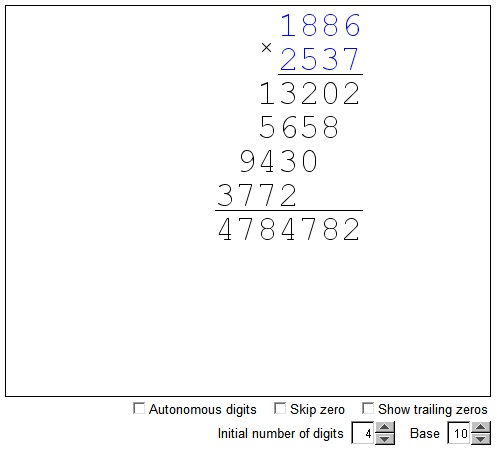# Long Multiplication

There are several multiplication algorithms, one - the Egyptian multiplication - came to us from antiquity and the same is probably true of the Vedic algorithm. Another method - the lattice multiplication - has been brought to Europe in the early 1200s, and the fourth one, known as the Russian Peasant multiplication, was in all likelihood developed much later and appeared in relatively modern times. A curious model of multiplication that was reputedly devised by a Chinese teacher has been making rounds on the Internet is probably not older than the Internet itself. Except of the Vedic variant, none claims a divine origin.

The multiplication scheme that everyone learns in school, the one that is often referred to as the traditional multiplication algorithm, is likely to have evolved from the lattice multiplication. As the Western world has been growing accustomed to the decimal system, so the arrangement of intermediate results became more compact and eventually formed a column of numbers whose sum gave the expected product. This, now indeed traditional, multiplication scheme is known as the long multiplication and in some place column multiplication.

The basis for the long multiplication is the distributive law. For example, to compute 23 × 46 we proceed as follows:

 23 × 46 = 23 × (40 + 6) = 23 × 40 + 23 × 6 = 920 + 138 = 1058.

The long multiplication is a particular arrangement of the numbers with alignment by the last digit, like this:

 2 3 × 4 6 1 3 8 9 2 0 1 0 5 8

In fact the second multiplicand is split from right to left, so that the multiplication by the a significant digit comes first. For example, for 23 × 761, we have

 23 × 761 = 23 × 1 + 23 × 60 + 23 × 700 = 23 + 1380 + 16100

which, according to the long multiplication scheme, is written as

 2 3 × 7 6 1 2 3 1 3 8 0 1 6 1 0 0 1 7 5 0 3

where, in the first row, 23 = 23 × 1, in the second row, 1380 = 23 × 60, and, in the third row, 16100 = 23 × 700. Customarily we use a more compact convention omitting the trailing zeros that are due to the powers of 10:

 2 3 × 7 6 1 2 3 1 3 8 1 6 1 1 7 5 0 3

The applet below offers an interactive version of the long multiplication. The two multiplicands appear in blue. There digits can be changed by dragging the cursor a little off center of each. The digits can change autonomously so that each will cycle through the values 1, 2, 3, ..., 0, or be made (the default) a part of the integer string so that, for example, if a digit changes from 9 to 0 its neighbor to the left accepts the carry of 1. The length of the multiplicands is controlled by the Initial number of digits spin, but also may change as the number itself changes.

### If you are reading this, your browser is not set to run Java applets. Try IE11 or Safari and declare the site https://www.cut-the-knot.org as trusted in the Java setup.What if applet does not run?### Related materialRead more...

• What Is Multiplication?
• Addition and Multiplication Tables in Various Bases
• Peasant Multiplication
• Egyptian Multiplication
• Lattice Multiplication - an Interactive Gizmo
• Group multiplication of permutations
• Using Math Rules: An Example
• Tables for Multiplication
•Copyright © 1996-2018 Alexander Bogomolny

 65264700